# Inverse Trigonometric Function

(redirected from Arctangent)
Also found in: Dictionary, Thesaurus, Acronyms, Wikipedia.

## inverse trigonometric function

[¦in‚vərs ‚trig·ə·nə‚me·trik ′fəŋk·shən]
(mathematics)
An inverse function of a trigonometric function; that is, an arc sine, arc cosine, arc tangent, arc cotangent, arc secant, or arc cosecant. Also known as antitrigonometric function.
McGraw-Hill Dictionary of Scientific & Technical Terms, 6E, Copyright © 2003 by The McGraw-Hill Companies, Inc.
The following article is from The Great Soviet Encyclopedia (1979). It might be outdated or ideologically biased.

## Inverse Trigonometric Function

a function that is a solution of the problem of finding an arc (number) from a given value of its trigonometric function. The six inverse trigonometric functions correspond to the six trigonometric functions: (1) Arc sin x, the inverse sine of x; (2) Arc cos x, the inverse cosine of x; (3) Arc tan x, the inverse tangent of x; (4) Arc cot x, the inverse cotangent of x; (5) Arc sec x, the inverse secant of x; and (6) Arc csc x, the inverse cosecant of x.

As an example, according to these definitions, x = Arc sin a is any solution of the equation sin x = a; that is, sin Arc sin a = a. The functions Arc sin x and Arc cos x are defined in the real domain for ǀxǀ ≤ 1, the functions Arc tan x and Arc cot x are defined for all real x, and the functions Arc sec x and Arc csc x are defined for ǀxǀ ≥ 1. The last two functions are rarely used.

Since trigonometric functions are periodic, their inverse functions are multiple-valued. Certain single-valued branches (the principal branches) of these functions are designated as arc sin x, arc cos x, …, arc csc x. Specifically, arc sin x: is the branch of the function Arc sin x for which –π/2 ≤ arc sin x ≤ π/2. Similarly, the functions arc cos x, arc tan x, and arc cot x are determined, respectively, from the conditions 0 < arc cos x ≤ π, –π/2 < arc tan x < π/2, and 0 < arc cot x < π. The inverse trigonometric functions Arc sin x, … can easily be expressed in terms of arc sin x, …; for example,

Arc sin x = (—1)n arc sin x + πn

Arc cos x = ±arc cos x + 2πn

Arc tan x = arc tan x + πn

Arc cot x = arc cot x + πn

where n = 0, ±1, ±2, ….

The well-known relations between the trigonometric functions yield relations between the inverse trigonometric functions, for example, the formula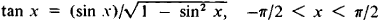implies that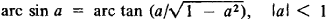The derivatives of the inverse trigonometric functions have the form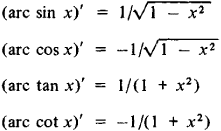The inverse trigonometric functions can be represented as power series, for example,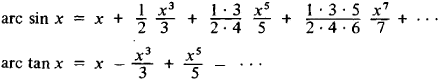These series converge for – 1 ≤ x ≤ 1.

The inverse trigonometric functions can be determined for arbitrary complex values of the independent variable, but the values of these functions will be real only for the values of the independent variable indicated above. Inverse trigonometric functions of a complex independent variable can be expressed by means of a logarithmic function, for example,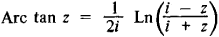### REFERENCE

Novoselov, S. I. Obratnye trigonometricheskie funktsii, 3rd ed. Moscow, 1950.
References in periodicals archive ?
This section presents similarity measures of NNs based on arctangent and tangent functions.
The vector direction (recommended angle of the vehicle by PFM or AFPFM) is calculated using the arctangent of the vector.
Thereby again two signals are generated, shifted by 90[degrees] - a sine and a cosine signal which enable an arctangent calculation to derive an absolute mechanic angle.
An arctangent function between [sigma] and T was found to be appropriate to consider the different effects of temperature on the end-to-end distances of the polymer chains at the glassy state, the glass transition and the rubbery state of the thermosets.
We then adopt the arctangent function to operate on the proportion , which can reduce the negative effect caused by noise.
RapidTables.com, Arctangent Calculator, http://www.rapidtables.com/calc/math/Arctan_Calculator.htm
The colour values were expressed in Hue angle (H[degrees]) and it calculated as arctangent ([b.sup.*]/[a.sup.*]) (0[degrees] = red-purple, 90[degrees] = yellow, 180[degrees] = bluish-green, 270[degrees] = blue).
We began our distance calculations by applying the arctangent of half the parallax angle of 22.83 arcseconds to half of the foreshortened baseline to estimate rough distances to Venus and the Sun.
In these imaging modalities and applications, the initial phases of the measured data are obtained by a simple operation of arctangent from the raw complex images and are wrapped in the principal interval (-[pi], [pi]].
The CORDIC with an orthogonal vector mode can compute the arctangent result iteratively [theta] = arctan(y/x), if the angle accumulator is initialized with zero ([z.sub.0] = 0).
By using the arctangent function property, it reduces the rotating judgment and add-subtract unit operation.

Site: Follow: Share:
Open / Close